## Series

#### Series

Direction: In the following questions two sets of pictures (Question Figure and Answer Figure) are given. In the Question Figure set, a series pattern (a), (b), (c), and (d) is given, you have to find out which picture from 1, 2, 3, and 4 of Answer Figure will continue the pattern.

1. Find out the next series from the Answer Figure that follows the sequence of the Question Figure.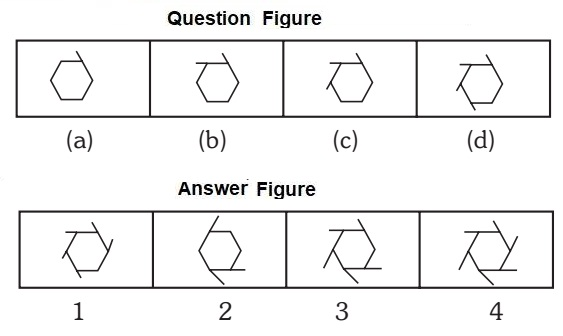1. Addition of line increases to the element.

##### Correct Option: C

Addition of line increases to the element.

1. Find out the next series from the Answer Figure that follows the sequence of the Question Figure.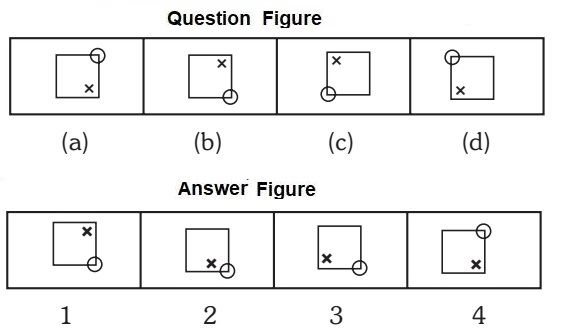1. The circle at the corner of the square moves clock wise, where as the cross inside the square moves anti clock wise.

##### Correct Option: D

The circle at the corner of the square moves clock wise, where as the cross inside the square moves anti clock wise.

1. Find out the next series from the Answer Figure that follows the sequence of the Question Figure.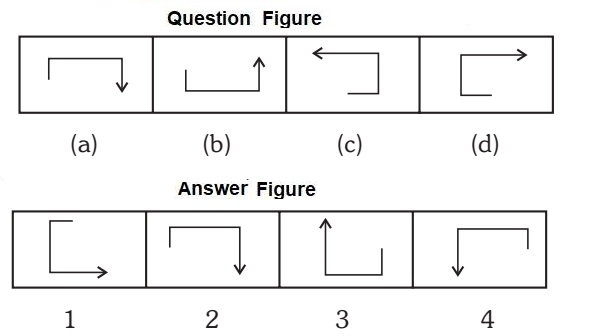1. In one step, the arrow and the small line segment turn to other side of the more line and in the next figure.

##### Correct Option: C

In one step, the arrow and the small line segment turn to other side of the more line and in the next figure.

1. Find out the next series from the Answer Figure that follows the sequence of the Question Figure.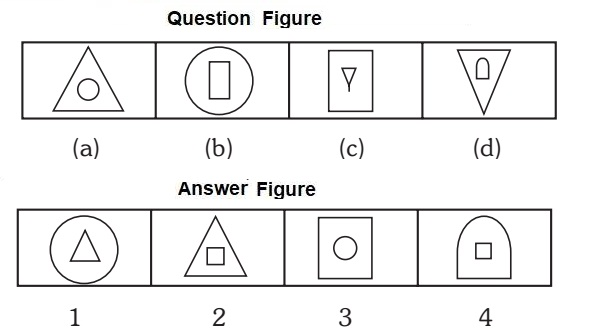1. Every time the inner figure enlarges and become the outer figure and the row inner figure changes to new element.

##### Correct Option: D

Every time the inner figure enlarges and become the outer figure and the row inner figure changes to new element.

1. Find out the next series from the Answer Figure that follows the sequence of the Question Figure.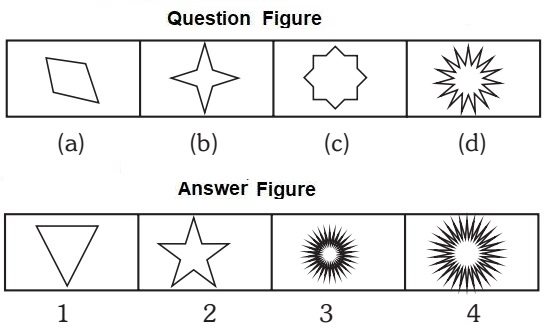1. The number of projections doubles in each step.

##### Correct Option: D

The number of projections doubles in each step.